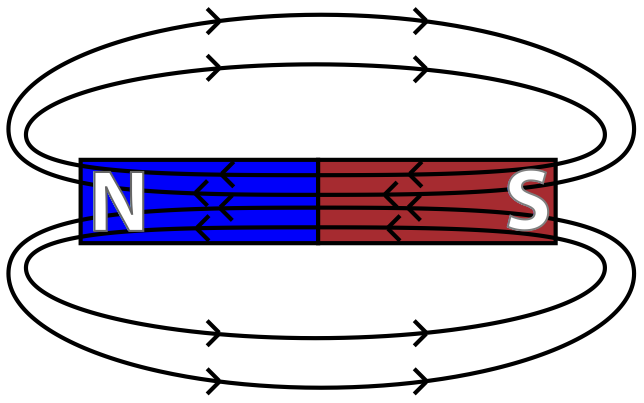# Magnetic FieldsMagnetism is closely related to electricity. In essence, magnetism is a force caused by moving charges. In the case of permanent magnets, the moving charges are the orbits of electrons spinning around nuclei. In very basic terms, strong permanent magnets have many atoms with electrons spinning in the same direction. Non-magnets have more random arrangements of electrons spinning around the nucleus. For electromagnets, the current itself provides the moving charges. In all cases, magnetic fields can be used to describe the forces due to magnets.

Question: Which type of field is present near a moving electric charge?

1. an electric field, only
2. a magnetic field, only
3. both an electric field and a magnetic field
4. neither an electric field nor a magnetic field

Answer: (3) An electric field is present due to the electric charge, and a magnetic field is present because the charge is in motion.

Magnets are polarized, meaning every magnet has two opposite ends. The end of a magnet that points toward the geographic north pole of the Earth is called the north pole of the magnet, while the opposite end, for obvious reasons, is called the magnet’s south pole. Every magnet has both a north and a south pole. There are no single isolated magnetic poles, or monopoles. If you split a magnet in half, each half of the original magnet exhibits both a north and a south pole, giving you two magnets. Physicists continue to search both physically and theoretically, but to date, no one has ever observed a north pole without a south pole, or a south pole without a north pole.

You used electric field lines to help visualize what would happen to a positive charge placed in an electric field. In order to visualize a magnetic field, you can draw magnetic field lines (also known as magnetic flux lines) which show the direction the north pole of a magnet would tend to point if placed in the field. Magnetic field lines are drawn as closed loops, starting from the north pole of a magnet and continuing to the south pole of a magnet. Inside the magnet itself, the field lines run from the south pole to the north pole. The magnetic field is strongest in areas of greatest density of magnetic field lines, or areas of the greatest magnetic flux density. Magnetic field strength (B) is measured in units known as Tesla (T).Much like electrical charges, like poles exert a repelling force on each other, while opposite poles exert an attractive force on each other. Materials can be classified as magnets, magnet attractables (materials which aren’t magnets themselves but can be attracted by magnets), and non-attractables.

Question: The diagram below shows the lines of magnetic force between two north magnetic poles. At which point is the magnetic field strength greatest?Answer: (B) has the greatest magnetic field strength because it is located at the highest density of magnetic field lines.

Question: The diagram below represents a 0.5-kilogram bar magnet and a 0.7-kilogram bar magnet with a distance of 0.2 meter between their centers.Which statement best describes the forces between the bar magnets?

1. Gravitational force and magnetic force are both repulsive.
2. Gravitational force is repulsive and magnetic force is attractive.
3. Gravitational force is attractive and magnetic force is repulsive.
4. Gravitational force and magnetic force are both attractive.

Answer: (3) Gravity always attracts and the north poles repel each other.

Question: A student is given two pieces of iron and told to determine if one or both of the pieces are magnets. First, the student touches an end of one piece to one end of the other. The two pieces of iron attract. Next, the student reverses one of the pieces and again touches the ends together. The two pieces attract again. What does the student definitely know about the initial magnetic properties of the two pieces of iron?

Answer: At least one of the pieces of iron is a magnet, but we cannot state with certainty that both are magnets.

Question: Draw a minimum of four field lines to show the magnitude and direction of the magnetic field in the region surrounding a bar magnet.

Answer:Question: When two ring magnets are placed on a pencil, magnet A remains suspended above magnet B, as shown at right. Which statement describes the gravitational force and the magnetic force acting on magnet A due to magnet B?

1. The gravitational force is attractive and the magnetic force is repulsive.
2. The gravitational force is repulsive and the magnetic force is attractive.
3. Both the gravitational force and the magnetic force are attractive.
4. Both the gravitational force and the magnetic force are repulsive.

Answer: (1) Gravity can only attract, and because magnet A is suspended above magnet B, the magnetic force must be repulsive.